# Solution assignment 01 Exponential functions and graphs

### Assignment 1

Draw the graph of the function: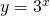What is the formula of the asymptote?

### Solution

The graph can be sketched using the most important and easiest point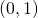. The graph can be sketched quite exact if we use a few other points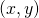, see the figure.The graph shows clearly that the-axis is an asymptote, so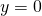. This can also be derived from the function: the more negativebecomes, the more the graph will approach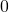.

0